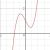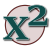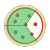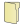Games
Problems
Go Pro!

# Factoring

Lesson Plans > Mathematics > Algebra > Factoring

# Featured Lesson PlansA worksheet involving factoring of polynomials with multiple variables, some using substitutionA lesson plan that leads students through a proof of the Quadratic FormulaHelping students have fun while factoring algebraic expressionsA different way of writing factor trees which may be helpful for algebra studentsA technique to help students prepare for simplifying algebraic fractionsLessons and teaching explanations for factoring trinomials - quadraticsDerivation of the quadratic formula - how to teach your students why this works# Full Directory Listing

Advanced Factoring Techniques, Equation Based Factor Trees, Factoring Trinomials (Quadratics) : Method With Examples, Fractions - Making Simplification Simple, Nicknames for Factoring RulesLike us on Facebook to get updates about new resources